# 2.3 Discrete Optimal Planning

This section extends Formulation 2.1 to allow optimal planning problems to be defined. Rather than being satisfied with any sequence of actions that leads to the goal set, suppose we would like a solution that optimizes some criterion, such as time, distance, or energy consumed. Three important extensions will be made: 1) A stage index will be used to conveniently indicate the current plan step; 2) a cost functional will be introduced, which behaves like a taxi meter by indicating how much cost accumulates during the plan execution; and 3) a termination action will be introduced, which intuitively indicates when it is time to stop the plan and fix the total cost.

The presentation involves three phases. First, the problem of finding optimal paths of a fixed length is covered in Section 2.3.1. The approach, called value iteration, involves iteratively computing optimal cost-to-go functions over the state space. Although this case is not very useful by itself, it is much easier to understand than the general case of variable-length plans. Once the concepts from this section are understood, their extension to variable-length plans will be much clearer and is covered in Section 2.3.2. Finally, Section 2.3.3 explains the close relationship between value iteration and Dijkstra's algorithm, which was covered in Section 2.2.1.

With nearly all optimization problems, there is the arbitrary, symmetric choice of whether to define a criterion to minimize or maximize. If the cost is a kind of energy or expense, then minimization seems sensible, as is typical in robotics and control theory. If the cost is a kind of reward, as in investment planning or in most AI books, then maximization is preferred. Although this issue remains throughout the book, we will choose to minimize everything. If maximization is instead preferred, then multiplying the costs by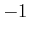and swapping minimizations with maximizations should suffice.

The fixed-length optimal planning formulation will be given shortly, but first we introduce some new notation. Let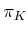denote a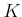-step plan, which is a sequence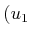,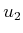,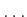,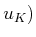ofactions. Ifand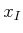are given, then a sequence of states,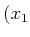,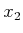,,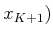, can be derived using the state transition function,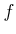. Initially,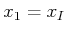, and each subsequent state is obtained by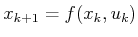.

The model is now given; the most important addition with respect to Formulation 2.1 is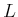, the cost functional.

Formulation 2..2 (Discrete Fixed-Length Optimal Planning)
1. All of the components from Formulation 2.1 are inherited directly:,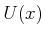,,, and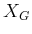, except here it is assumed thatis finite (some algorithms may easily extend to the case in whichis countably infinite, but this will not be considered here).
2. A number,, of stages, which is the exact length of a plan (measured as the number of actions,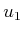,,,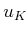). States may also obtain a stage index. For example,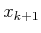denotes the state obtained after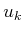is applied.
3. Letdenote a stage-additive cost (or loss) functional, which is applied to a-step plan,. This means that the sequence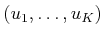of actions and the sequence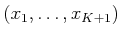of states may appear in an expression of. For convenience, let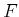denote the final stage,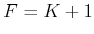(the application ofadvances the stage to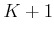). The cost functional is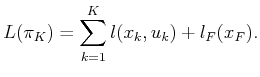(2.4)

The cost term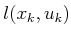yields a real value for every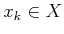and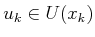. The final term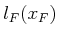is outside of the sum and is defined as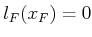if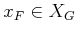, and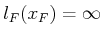otherwise.

An important comment must be made regarding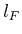. Includingin (2.4) is actually unnecessary if it is agreed in advance thatwill only be applied to evaluate plans that reach. It would then be undefined for all other plans. The algorithms to be presented shortly will also function nicely under this assumption; however, the notation and explanation can become more cumbersome because the action space must always be restricted to ensure that successful plans are produced. Instead of this, the domain ofis extended to include all plans, and those that do not reachare penalized with infinite cost so that they are eliminated automatically in any optimization steps. At some point, the role ofmay become confusing, and it is helpful to remember that it is just a trick to convert feasibility constraints into a straightforward optimization (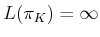means not feasible and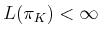means feasible with cost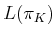).

Now the task is to find a plan that minimizes. To obtain a feasible planning problem like Formulation 2.1 but restricted to-step plans, let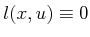. To obtain a planning problem that requires minimizing the number of stages, let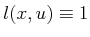. The possibility also exists of having goals that are less crisp'' by letting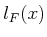vary for different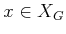, as opposed to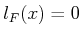. This is much more general than what was allowed with feasible planning because now states may take on any value, as opposed to being classified as inside or outside of.

Subsections
Steven M LaValle 2020-08-14